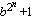# generalized Fermat prime

Any prime generalized Fermat number Fb,n =(with b an integer greater than one) is called a generalized Fermat prime (because they are Fermat primes in the special case b=2).

Why is the exponent a power of two? Because if m is an odd divisor of n, then bn/m+1 divides bn+1, so for the latter to be prime, m must be one.  Because the exponent is a power of two, it seems reasonable to conjecture that the number of Generalized Fermat primes is finite for every fixed base b.